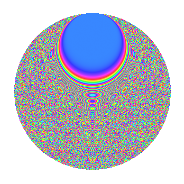# Properties

 Label 2365.2.brLevel 2365 Weight 2 Character orbit br Rep. character $$\chi_{2365}(173,\cdot)$$ Character field $$\Q(\zeta_{20})$$ Dimension 2016 Sturm bound 528

# Related objects

## Defining parameters

 Level: $$N$$ = $$2365 = 5 \cdot 11 \cdot 43$$ Weight: $$k$$ = $$2$$ Character orbit: $$[\chi]$$ = 2365.br (of order $$20$$ and degree $$8$$) Character conductor: $$\operatorname{cond}(\chi)$$ = $$55$$ Character field: $$\Q(\zeta_{20})$$ Sturm bound: $$528$$

## Dimensions

The following table gives the dimensions of various subspaces of $$M_{2}(2365, [\chi])$$.

Total New Old
Modular forms 2144 2016 128
Cusp forms 2080 2016 64
Eisenstein series 64 0 64

## Trace form

 $$2016q - 4q^{3} + O(q^{10})$$ $$2016q - 4q^{3} + 16q^{11} - 64q^{12} - 40q^{15} + 504q^{16} - 76q^{20} - 28q^{22} - 16q^{23} - 36q^{25} - 48q^{26} - 4q^{27} - 120q^{28} - 24q^{33} + 344q^{36} + 4q^{37} - 120q^{41} - 40q^{42} - 16q^{45} + 20q^{47} + 40q^{48} - 80q^{51} + 40q^{53} + 84q^{55} + 8q^{56} - 80q^{57} + 48q^{58} - 136q^{60} + 80q^{61} - 300q^{62} + 44q^{66} - 80q^{67} - 40q^{70} - 104q^{71} - 300q^{72} - 120q^{73} - 80q^{75} - 164q^{77} - 112q^{78} - 120q^{80} + 392q^{81} - 32q^{82} - 200q^{83} - 80q^{85} - 108q^{88} - 160q^{90} - 96q^{91} + 196q^{92} - 108q^{93} - 180q^{96} - 68q^{97} + O(q^{100})$$

## Decomposition of $$S_{2}^{\mathrm{new}}(2365, [\chi])$$ into newform subspaces

The newforms in this space have not yet been added to the LMFDB.

## Decomposition of $$S_{2}^{\mathrm{old}}(2365, [\chi])$$ into lower level spaces

$$S_{2}^{\mathrm{old}}(2365, [\chi]) \cong$$ $$S_{2}^{\mathrm{new}}(55, [\chi])$$$$^{\oplus 2}$$

## Hecke Characteristic Polynomials

There are no characteristic polynomials of Hecke operators in the database